Your research can change the world
More on impact ›

# Frontiers in Mechanical Engineering## ORIGINAL RESEARCH article

Front. Mech. Eng., 12 June 2020 | https://doi.org/10.3389/fmech.2020.00041

# Analysis of Energy Transport Behavior and Geometric Effects in Graphene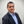Alejandro Guajardo-Cuéllar1*,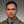David B. Go2,3 andMihir Sen2
• 1Escuela de Ingenieria y Ciencias, Tecnologico de Monterrey, Zapopan, Mexico
• 2Department of Aerospace and Mechanical Engineering, University of Notre Dame, Notre Dame, IN, United States
• 3Department of Chemical and Biomolecular Engineering, University of Notre Dame, Notre Dame, IN, United States

Graphene is an excellent heat conductor, with the potential to be used as a heat spreader for applications where there are fast, transient heat pulses. In this study we analyze and describe energy transport in graphene subject to an initial pulse of energy. We analyze the effects of using harmonic, anharmonic, and a non-linear (Tersoff) potentials to describe the transient energy transport and compare these to classical continuum descriptions. The energy pulse produces pure wave-like behavior and a spatial energy distribution that has geometric features similar to the graphene geometry itself. Depending on the potential used, the energy travels outward from the impulse location following a similar pattern as the hexagonal shape of graphene. This pattern is clearly identified when the transport is treated with a harmonic potential. Increasing the anharmonicity and non-linearity dampens this effect and results in thermal transport that does not follow the geometry of graphene.

## 1. Introduction

During the last 30 years there has been an exponential growth of devices and technologies built from materials at the nanoscale, in large part a result of the advances in transistors predicted by Moore (1965). Discovered by Novoselov et al. (2004), graphene has been shown to have excellent electrical properties including an extremely high electron mobility (Geim and Novoselov, 2007), and is thus a promising material to continue this growth. It also has good mechanical properties including a large Young's modulus that makes it a rigid and strong material (Frank et al., 2007). These properties, as well as favorable optical and magnetic properties (Geim and Novoselov, 2007; Kuzmenko et al., 2008; Nair et al., 2008), open a large window of possibilities for the exploration of new graphene-based materials and devices.

The thermal properties of graphene are particularly exceptional. Balandin et al. (2008) reported the first experimental value for the thermal conductivity, approximately 5,000 W m−1 K−1. This extremely high number opened a new field of study to measure and to calculate the thermal conductivity. Reported results show that the value can vary from 500 W m−1 K−1 (Cai et al., 2010) up to above 5,000 W m−1 K−1 (Nika et al., 2009a,b; Evans et al., 2010; Aksamija and Knezevic, 2011). The reasons behind such large range of values have been widely discussed. Nika and Balandin (2012) reviewed the values and reported that differences in fabrication methods, sample size, measuring technique, temperature and boundary conditions produce different results. The variation among results helped guide investigations into which factors impact the thermal transport. Calculations of the thermal conductivity from theoretical models and computational techniques provided similar conclusions as the measurements. Seol et al. (2010) reported a value of ≈ 3,000 W m−1 K−1. The value is calculated for free graphene at room temperature. This condition is similar as the case we study here. The value is extremely high value compared with metals. This property, in particular, makes graphene attractive for thermal management technologies, such as heat spreaders in electronics and other applications (Pop et al., 2012; Cahill et al., 2014).

Despite the interest and broad study of energy transport in graphene in the past decade, there is still not a consensus on the characteristics of phonons traveling in graphene, and specifically whether the transport is ballistic or exhibits normal or anomalous diffusive behavior. A comparison of the mean free path (MFP) of the phonons in graphene (Ghosh et al., 2008) with the dimensions of the structure has been reported and provides relevant information about ballistic transport or scattering effects. In a complete review on the topic of anomalous diffusion in graphene, Liu et al. (2012) described investigations where anomalous diffusion is observed, specifically molecular dynamics simulations and experimental measurements where the thermal conductivity is size dependent. It is generally understood that for dimensions smaller than the mean free path of the phonons, the thermal conductivity is size dependent. Mu et al. (2015) studied coherent and incoherent phonon transport in modified graphene lattices. They also observed length dependence of the thermal conductivity in the super lattice. In this same study, they reported the relation of coherent phonons with ballistic transport and the crossover from coherent phonons to incoherent phonons in terms of length.

In contrast to these studies, Saito et al. (2018) proposed that the transport is ballistic or diffusive as a function of temperature and size, using a Hamiltonian that included third-order terms. They reported that the crossover from ballistic to diffusive occurs at about 100 K, where the thermal conductivity becomes size dependent. Above 400 K, however, the thermal conductivity did not change for differently-sized samples. Behavior other than diffusive or ballistic in graphene has also been reported, such as the hydrodynamic behavior of phonons resembling Poiselle flow (Lee et al., 2015).

Maassen and Lundstrom (2015) developed a model similar to electron transport theory for phonons. They reported that they obtained equivalent results from using Fourier's Law and the Boltzmann transport equation for ballistic transport. Steady state ballistic transport can be captured using Fourier's law and proper boundary conditions (Kaiser et al., 2017), but the method is limited to steady state without generation terms. Thermoreflectance at the boundaries with periodic heat generation in the boundary for ballistic transport can also be modeled using an approach developed by Maassen and Lundstrom (2016).

The identification of different behaviors or regimes of transport based on conditions such as size, defects, boundary conditions, and how the graphene is mounted, either isolated or on a substrate, has also motivated investigations into how to design graphene with specific thermal characteristics. For example, Mu et al. (2014) reported the effects of oxidation on the thermal transport, showing that ballistic transport or scattering can be tuned depending upon the level of oxidation.

Here we analyze how graphene responds to a pulse of thermal energy, using different inter-atomic potentials and comparison to continuum models to differentiate thermal behavior. In order to determine if the behavior is diffusive, wave-like, or something else, we initially perturb a single atom in a graphene sheet and monitor its temporal response. We evaluate the autocorrelation of the energy and compare it to solutions to the diffusion and wave equation. We then also monitor the temporal evolution of the spatial distribution of the energy pulse throughout the graphene sheet. We observe interesting geometric behavior that reflects the hexagonal shape of graphene, though this depends strongly on the nature of the inter-atomic behavior. The findings we present shed additional insight into the behavior of thermal transport in graphene, and further inform how to design and optimize graphene for applications such as heat spreaders.

## 2. Methods

### 2.1. Model System

We study a single graphene sheet with 1,024 atoms and dimensions of approximately 40 by 70 Å as a model system. The length scale of the graphene sheet is at least one order of magnitude smaller than the mean free path of phonons in single layer graphene as reported by different authors (Ghosh et al., 2008; Gholivand and Donmezer, 2017). The graphene structure is two dimensional (2-d) and is fixed in space. Each atom in the sheet is allowed to move in the x, y, and z directions. The dynamics of the atoms in the crystal lattice were solved numerically, and each atom was bonded to its closest neighbors through an inter-atomic potential, U, with periodic boundary conditions applied at the edges of the sheet. The derivative of the potential with respect to the position is the force on the atom, F = −∇U. The position and the velocity of each atom in the system is solved in time by integrating the force on each atom using the Gear-predictor numerical scheme, and then the kinetic energy of each individual atom is calculated.

Phonons are bosons that observe the Bose-Einstein distribution. Here we use equilibrium molecular dynamics EMD, a classical mechanics approach that does not consider quantum effects. Fan et al. (2019) introduced quantum effects in the parameters of the Tersoff potential after doing first principles calculations. They report that quantum effects below the Debye temperature, as calculated here, are not negligible but small; they affect about 10 % of the final value in the calculation of the thermal conductivity. In the next sections we compare the results of EMD with continuum models. The description here considers only the energy transport contribution from a classical mechanical description. The modeling used does not describe the small contribution of the quantum effects of phonons.

The basic strategy is to analyze the time variation of the spatial distribution of kinetic energy throughout the graphene sheet. An initial perturbation in velocity equally shared in all three dimensions is given to the atom at the middle of the structure, while all other atoms in the sheet were set in their equilibrium position with a zero initial velocity. The initial condition given to the system is an initial velocity to a central atom, i.e., at a temperature greater than 0 K and the rest of the atoms with no kinetic energy (effectively 0 K). At time t = 0 all the energy in the system is contained solely in the perturbed atom, and the rest of the atoms are in equilibrium with no energy. The initial position of the disturbed atom is the equilibrium position and the initial velocity has a magnitude of 1.73 $\frac{Å}{ps}$. The magnitude corresponds to an initial velocity in each direction with a value of 1 $\frac{Å}{ps}$. For times t > 0, the energy is transferred from the central atom to its neighboring atoms and so on throughout the structure. This evolution is shown in Figure 1, where the distribution of kinetic energy for two different times is shown. Figure 1A shows the initial distribution of kinetic energy and Figure 1B shows the distribution for time t = 3 ps where the initial peak at the center of the lattice has decreased and additional peaks appear as the energy spreads.

FIGURE 1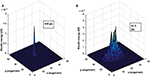Figure 1. Kinetic energy distribution at time t = 0 ps for an initial energy of 19 ×10−4 eV using the harmonic potential. (A) t = 0 ps and (B) t = 3 ps.

### 2.2. Inter-Atomic Potentials

Two different artificial potentials and then a single more realistic potential are used here. The first artificial potential is a harmonic potential, which in 1-d is defined as

where k1 is a spring constant reflecting the inter-atomic bond and xi is the displacement of the spring attached to atom i. The second artificial potential is an anharmonic potential, which includes an anharmonic term with spring constant k2 as

Both of these potentials are used in order to generically analyze the effect of non-linearity in the inter-atomic potential on energy transport. Also, both potentials just consider nearest neighbor interactions.

Equivalent values for the spring constants k1 and k2 are obtained by using the lower order terms of the Taylor series expansion of the Lennard-Jones (LJ) potential for carbon (Girifalco and Lad, 1956; Rafati et al., 2010). The values for k1 and k2 are k1 = 0.1922 N/m and k2 = 0.5291 N/m, respectively, and the ratio is k2/k1=2.75. The LJ potential models weak interactions within atoms. These potentials represent the atomic vibrations resulting from weak interactions and missing the primary bonds. The geometry is graphene but the potential does not represent the complete interatomic bonding.

We compare these two artificial potentials to the Tersoff potential (Tersoff, 1988b, 1989), which more accurately reflects the inter-atomic bonding in graphene (Tersoff, 1988a; Berber et al., 2000; Guajardo-Cuéllar et al., 2010; Lindsay and Broido, 2010; Singh et al., 2011; Rajasekaran et al., 2016). Since the Tersoff potential is significantly more complex, comparison to these simpler potentials allows us to identify harmonic and anharmonic behaviors.

The Tersoff potential not only accounts for the interaction between atom i and its nearest neighbor j, but also the interaction of i with all its neighbors k, where the covalent bonds maintain the geometry of the crystal. The potential is given by

Here, fR(rij) is a repulsive term, fA(rij) is an attractive term, and fC(rij) is a cut-off function, where rij is the distance between atoms i and j. The parameter γij is a multi-body term that accounts for atom k and ensures that when the two-body interaction between atom i and atom j is calculated, the rest of the atoms of the system are not ignored. That is, the Tersoff potential is a two-body potential that takes into account the non-local environment (atom k) when defining the local potential energy between atom i and atom j. The parameters are given by Tersoff (1989) for carbon, where the values of the parameters were obtained from a fitting process of theoretical calculations.

## 3. Results

### 3.1. Wave-Like vs. Diffusive Transport Behavior

In order to analyze our simulations of the dynamics in the graphene sheet, we compare the behavior to classical wave and diffusive transport by analyzing a 2-d sheet using continuum expressions.

In 2-d, the wave equation is

where C is a transport quantity that for the purpose of this investigation is kinetic energy, and v is the wave speed. Similarly, the 2-d diffusion equation is

where D is the diffusivity coefficient. A unit value is used for the wave speed and the diffusivity. The value is adequate to capture the qualitative characteristics of each behavior. For the wave speed, the value leads to results that are on the same order of magnitude for the wave speed and the dimensions of the sample. The same criteria is used for the diffusivity, where a unit value leads to the proper time scale for the transient behavior and a good comparison within the same time scale as the motion of each individual atom.

Similar to our treatment of the graphene sheet, periodic boundary conditions are imposed for both equations

where Lx is the length in the x direction and Ly is the length in the y direction. To simulate an impulse of energy on a single atom in the graphene sheet, an initial condition is imposed at the middle of the domain

where x0 = Lx/2, and y0 = Ly/2. We solve both the 2-d wave and diffusion equations numerically using the finite difference method, and have confirmed convergence of the solution. The convergence is verified via energy conservation of the initial pulse during the entire time of the solution. The time step for the finite differences is 6.6 × 10−5 arbitrary units (au), and with this time step stability and energy conservation is observed. The integration for the equations of motion for the EMD simulations used an integration time step of 1 fs for the harmonic and anharmonic potentials, which was sufficient to avoid numerical diffusion. A time step of 0.5 fs was used for the Tersoff potential, which was required to keep the scheme stable. The time units compare well for both the finite difference and EMD methods as the time step is four order of magnitude smaller than the time domain of interest. The maximum time studied in the continuum descriptions is 0.2 arbitrary units with a time step four orders of magnitude smaller. The time domain of interest for the EMD is 40 ps with a time step of 1 fs, which is also four orders of magnitude difference.

We use the quantity C2(x0, y0) at the center of our domain to compare the results of the continuum models to the normalized kinetic energy of the central, perturbed atom from our molecular dynamics simulations. As an example, Figure 2 shows the temporal evolution from the solution of the 2-d wave and diffusion equations. It is clear the two behaviors are distinct. For diffusion, the energy spreads uniforms away from the center of the domain (x0, y0) such that the quantity C2 decays constantly (dotted line). In the graph C2 is presented in logarithmic scale and as expected the exponential decay is linear. In contrast, the wave equation results in a wave-like, oscillatory behavior of the transport quantity C2.

FIGURE 2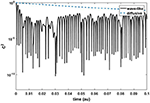Figure 2. Temporal evolution of the quantity C2(x0, y0) for the wave equation Equation (4) (solid line) and for the diffusion equation Equation (5) (dotted line).

For comparison, Figure 3 shows the time evolution of the normalized kinetic energy of central atom in the lattice for the harmonic (Figure 3A), anharmonic (Figure 3B), and Tersoff (Figure 3C) inter-atomic potentials. In the wave equation solution shown in Figure 2, the magnitude of the solution decays with oscillatory behavior until approximately t = 0.03 au (arbitrary units), then the magnitude grows for approximately t = 0.02 au before falling suddenly and repeating this behavior. This is the result of the wave traveling through the periodic structure. A similar effect is observed in the simulation of kinetic energy using the harmonic potential, Figure 3A. The energy decays for the initial 20 ps, then energy starts recovering amplitude, and wave-like behavior is again observed after 30 ps. The harmonic potential results in behavior similar to the wave equation since it represents a perfect harmonic oscillator.

FIGURE 3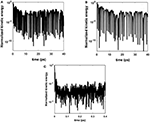Figure 3. Kinetic energy atom 544 in graphene. (A) Harmonic potential, (B) anharmonic potential, and (C) Tersoff potential.

When adding non-linearity through anharmonic terms, the same general wave-like transport is still observed as shown in Figure 3B. Even more surprising, when using the Tersoff potential, which adds more severe non-linearity, the same oscillatory, wave-like behavior also occurs, Figure 3C, but the time timescale of the initial decay is far faster than the decay for the harmonic and anharmonic potentials. Figure 3C shows the normalized kinetic energy over the first 1 ps and the initial wave decays really fast, in less than 10% of 1 ps. This is approximately two orders of magnitude faster than the harmonic and anharmonic potentials, and could possibly be attributed to the multi-body terms in the Tersoff potential increasing damping in the energy. The qualitative comparison between the continuum equations and the dynamics simulations confirm that for this periodic graphene structure, the energy transport from an initial energy pulse takes on wave-like behavior.

Another way of analyzing the behavior is using the autocorrelation function. Autocorrelation analysis compares a signal in time with the same signal after some time lag τ. Mathematically this is defined as

where Δt is a time delay from the origin. Figure 4 shows the autocorrelation function of C for the wave and the diffusion Equations (4) and (5). Again there is a notable difference; the autocorrelation of the wave equation decays steadily, but for the diffusion equation it initially decays and then recovers.

FIGURE 4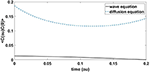Figure 4. Evolution of the autocorrelation of C for the diffusion (dash) and wave (solid) Equations (4) and (5).

For comparison, Figure 5 shows the autocorrelation of the normalized kinetic energy for the central atom in the lattice for the (Figure 5A), anharmonic (Figure 5B), and Tersoff (Figure 5C) potentials. In all cases, the autocorrelation is quickly damped, with much longer time scales for the harmonic and anharmonic potentials (τ ~ 10 ps) relative to the Tersoff potential. This is consistent with the wave equation shown in Figure 4, with the major exception that the autocorrelation is much noisier from the dynamics simulations. In particular, the Tersoff potential produces an autocorrelation that damps within 1 ps but is noisier than either the harmonic or anharmonic.

FIGURE 5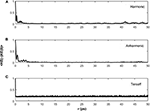Figure 5. Autocorrelation function of the normalized kinetic energy of the central atom in the graphene lattice for the (A) harmonic, (B) anharmonic, and (C) Tersoff potentials.

Both the magnitude analysis and autocorrelation analysis suggest that even though there is significant non-linearity in the Tersoff potential, the energy transport behavior in a graphene sheet will still have wave-like features when subjected to a localized energy pulse. To understand this wave behavior more, we analyzed the power spectral density (PSD) of the signal. The PSD is calculated in Fourier space by

where $\stackrel{^}{KE}\left(\omega \right)$ is the kinetic energy in frequency space, and $\stackrel{^}{KE}{\left(\omega \right)}^{*}$ is the complex conjugate. The components of frequency for the harmonic potential are shown in Figure 6A. Using this potential, there are low frequency components up to 2.5 THz. The anharmonic potential also produces low frequency components as shown in Figure 6B with only small qualitatively different features from the harmonic potential. The frequencies are lower than the reported values for graphene (Taheri et al., 2018). As discussed previously the harmonic and anharmonic potential used here do not model the primary atomic bonds; the spectra observed are from the vibrations of atoms due to weak interatomic bonding. The Tersoff potential, in contrast, produces frequencies an order of magnitude larger than the harmonic and the anharmonic potentials, with component frequencies up to 35 THz. Similar values for the Tersoff potential spectra have been reported (Nika and Balandin, 2012; Taheri et al., 2018). Further, there are three distinct frequencies at ≈ 4.8, ≈ 27, and ≈ 33 THz, which suggest that oscillations at these frequencies dominate the wave-like behavior.

FIGURE 6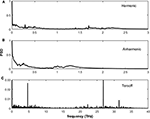Figure 6. Power spectral density of kinetic energy of the central atom in the graphene lattice for the (A) harmonic, (B) anharmonic, and (C) Tersoff potentials.

### 3.2. Geometric Behavior

While the above analysis focused solely on the energy of the perturbed atom in the graphene lattice, analyzing the spatial evolution of the energy also reveals important facets of the energy transport. Figure 7 shows snapshot contours of the kinetic energy for the entire lattice. When using the harmonic potential, the energy is initially concentrated at the center at 0.02 ps immediately after the initial pulse (Figure 7a) and then spreads radially, creating a profile that reflects the hexagonal structure of the graphene lattice (rotated 30°) by 0.28 ps (Figure 7b). Similarly, for the anharmonic potential, the profile from the initial energy pulse at 0.02 ps (Figure 7c) to 0.28 ps (Figure 7d) also produces a clear hexagonal shape.

FIGURE 7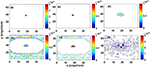Figure 7. Contour lines of kinetic energy distribution: (a) harmonic potential at 0.02 ps, (b) harmonic potential at 0.28 ps, (c) anharmonic potential at 0.02 ps, (d) anharmonic potential at 0.28 ps, (e) Tersoff potential at 0.02 ps, and (f) Tersoff potential at 0.28 ps.

This geometric behavior suggest that the waves propagate in an ordered fashion, where the geometry of the lattice plays a significant role in the energy transport. However, the non-linearity and multi-body terms in the Tersoff potential disrupt this behavior, as shown in Figures 7e,f. As the energy spreads, the hexagonal shape is not observed, and behavior appears more diffuse. Thus, while the behavior of a single atom has wave-like attributes, when the whole geometry of the 2D lattice is considered, the wave propagation is much more complex.

Previously, wave-like behavior was identified in Figure 6. The wave-like behavior energy transport is not evident using the Tersoff potential in Figures 7e,f. (Dong et al., 2014) conducted a study for ripple propagation in 2-d graphene. The initial condition of their study is an initial energy perturbation after a C60 molecule hits the graphene sheet. For pristine graphene, they observe a disordered distribution of energy after the initial disturbance, similar as what is observed here. The energy distribution observed in Dong et al. (2014) is the result of reflection of the ripples from the fixed edges. Here, the traveling wave comes back to the initial perturbed atom once it travels through the domain due to the periodic boundary conditions. The wave interacts with the instant vibration of the atom, a similar effect as reflection. Consequently, we cannot distinguish wave behavior in the energy distribution.

## 4. Discussion and Conclusions

In this work, we investigated the transport of a single, discrete pulse of thermal energy through a periodic graphene sheet. By evaluating different atomic potentials and comparing the simulated behavior to classical wave and diffusion expressions, we found that the thermal transport has significant wave-like characteristics, which we owe to the fact that the dimensions of the graphene sheet studied here are smaller than the mean free path of phonons in graphene. With different sizes, we might observe a delay in the scattering observed from the initial traveling wave reaching the location of the initial perturbation. The results report pure wave-like behavior. The characteristics for the transport studied here are not expected to vary with different sizes of the graphene sheet. However, when comparing the realistic Tersoff potential to model harmonic and anharmonic potentials, it is clear that multi-body effects accelerate the thermal transport, with characteristic times about an order of magnitude smaller for every characterized parameter (amplitude, autocorrelation, and geometric behavior). Interestingly, when the potential is harmonic or only weakly anharmonic, the wave propagates through the graphene sheet along the bonds of the atoms, forming a hexagonal pattern that resembles the honeycomb structure of graphene. The greater anharmonicity and multi-body interactions introduced by the Tersoff potential do not show this geometric behavior, and although the transport still retains wave-like qualities, diffusive aspects also appear.

## Data Availability Statement

The datasets generated for this study are available on request to the corresponding author.

## Author Contributions

All the authors developed the theory and methods, discussed the results, and contributed to the final manuscript. AG–C performed the computations.

## Conflict of Interest

The authors declare that the research was conducted in the absence of any commercial or financial relationships that could be construed as a potential conflict of interest.

## References

Aksamija, Z., and Knezevic, I. (2011). Lattice thermal conductivity of graphene nanoribbons: anisotropy and edge roughness scattering. Appl. Phys. Lett. 98:141919. doi: 10.1063/1.3569721

Balandin, A. A., Ghosh, S., Bao, W., Calizo, I., Teweldebrhan, D., Miao, F., et al. (2008). Superior thermal conductivity of single-layer graphene. Nano Lett. 8, 902–907. doi: 10.1021/nl0731872

Berber, S., Kwon, Y.-K., and Tománek, D. (2000). Unusually high thermal conductivity of carbon nanotubes. Phys. Rev. Lett. 84, 4613–4616. doi: 10.1103/PhysRevLett.84.4613

Cahill, D. G., Braun, P. V., Chen, G., Clarke, D. R., Fan, S., Goodson, K. E., et al. (2014). Nanoscale thermal transport. II. 2003–2012. Appl. Phys. Rev. 1:011305. doi: 10.1063/1.4832615

Cai, W., Moore, A., Zhu, Y., Li, X., Chen, S., Shi, L., et al. (2010). Thermal transport in suspended and supported monolayer graphene grown by chemical vapor deposition. Nano Lett. 10, 1645–1651. doi: 10.1021/nl9041966

Dong, Y., He, Y., Wang, Y., and Li, H. (2014). A theoretical study of ripple propagation in defective graphene. Carbon 68, 742–747. doi: 10.1016/j.carbon.2013.11.060

Evans, W. J., Hu, L., and Keblinski, P. (2010). Thermal conductivity of graphene ribbons from equilibrium molecular dynamics: effect of ribbon width, edge roughness, and hydrogen termination. Appl. Phys. Lett. 96:203112. doi: 10.1063/1.3435465

Fan, Z., Wang, Y., Gu, X., Qian, P., Su, Y., and Ala-Nissila, T. (2019). A minimal tersoff potential for diamond silicon with improved descriptions of elastic and phonon transport properties. J. Phys. Condensed Matter 32:135901. doi: 10.1088/1361-648X/ab5c5f

Frank, I. W., Tanenbauma, D. M., van der Zande, A. M., and McEuen, P. L. (2007). Mechanical properties of suspended graphene sheets. J. Vacuum Sci. Technol. B 25, 2558–2561. doi: 10.1116/1.2789446

Geim, A., and Novoselov, K. (2007). The rise of graphene. Nat. Mater. 6, 183–191. doi: 10.1038/nmat1849

Gholivand, H., and Donmezer, N. (2017). Phonon mean free path in few layer graphene, hexagonal boron nitride, and composite bilayer H-BN/graphene. IEEE Trans. Nanotechnol. 16, 752–758. doi: 10.1109/TNANO.2017.2672199

Ghosh, S., Calizo, I., Teweldebrhan, D., Pokatilov, E. P., Nika, D. L., Balandin, A. A., et al. (2008). Extremely high thermal conductivity of graphene: prospects for thermal management applications in nanoelectronic circuits. Appl. Phys. Lett. 92:151911. doi: 10.1063/1.2907977

Girifalco, L., and Lad, R. (1956). Energy of cohesion, compressibility, and the potential energy functions of the graphite system. J. Chem. Phys. 25, 693–697. doi: 10.1063/1.1743030

Guajardo-Cuéllar, A., Go, D. B., and Sen, M. (2010). Evaluation of heat current formulations for equilibrium molecular dynamics calculations of thermal conductivity. J. Chem. Phys. 132:104111. doi: 10.1063/1.3358338

Kaiser, J., Feng, T., Maassen, J., Wang, X., Ruan, X., and Lundstrom, M. (2017). Thermal transport at the nanoscale: A Fourier's law vs. phonon Boltzmann equation study. J. Appl. Phys. 121:044302. doi: 10.1063/1.4974872

Kuzmenko, A., van Heumen, E., Carbone, F., and van der Marel, D. (2008). Universal optical conductance of graphite. Phys. Rev. Lett. 100:117401. doi: 10.1103/PhysRevLett.100.117401

Lee, S., Broido, D., Esfarjani, K., and Chen, G. (2015). Hydrodynamic phonon transport in suspended graphene. Nat. Commun. 6:6290. doi: 10.1038/ncomms7290

Lindsay, L., and Broido, D. A. (2010). Optimized tersoff and brenner empirical potential parameters for lattice dynamics and phonon thermal transport in carbon nanotubes and graphene. Phys. Rev. B 81:205441. doi: 10.1103/PhysRevB.81.205441

Liu, S., Xu, X., Xie, R., Zhang, G., and Li, B. (2012). Anomalous heat conduction and anomalous diffusion in low dimensional nanoscale systems. Eur. Phys. J. B 85:337. doi: 10.1140/epjb/e2012-30383-8

Maassen, J., and Lundstrom, M. (2015). Steady-state heat transport: ballistic-to-diffusive with Fourier's law. J. Appl. Phys. 117:035104. doi: 10.1063/1.4905590

Maassen, J., and Lundstrom, M. (2016). Modeling ballistic effects in frequency-dependent transient thermal transport using diffusion equations. J. Appl. Phys. 119:095102. doi: 10.1063/1.4942836

Moore, G. (1965). Cramming more components onto integrated circuits. Electronics 38:114–117.

Mu, X., Wu, X., Zhang, T., Go, D. B., and Luo, T. (2014). Thermal transport in graphene oxide from ballistic extreme to amorphous limit. Sci. Rep. 4:3909. doi: 10.1038/srep03909

Mu, X., Zhang, T., Go, D. B., and Luo, T. (2015). Coherent and incoherent phonon thermal transport in isotopically modified graphene superlattices. Carbon 83, 208–216. doi: 10.1016/j.carbon.2014.11.028

Nair, R., Blake, P., Grigorenko, A., Novoselov, K., Booth, T., Stauber, T., et al. (2008). Fine structure constant defines visual transparency of graphene. Science 320:1308. doi: 10.1126/science.1156965

Nika, D. L., and Balandin, A. A. (2012). Two-dimensional phonon transport in graphene. J. Phys. Condensed Matter 24:233203. doi: 10.1088/0953-8984/24/23/233203

Nika, D. L., Ghosh, S., Pokatilov, E. P., and Balandin, A. A. (2009a). Lattice thermal conductivity of graphene flakes: comparison with bulk graphite. Appl. Phys. Lett. 94:203103. doi: 10.1063/1.3136860

Nika, D. L., Pokatilov, E. P., Askerov, A. S., and Balandin, A. A. (2009b). Phonon thermal conduction in graphene: role of Umklapp and edge roughness scattering. Phys. Rev. B 79:155413. doi: 10.1103/PhysRevB.79.155413

Novoselov, K., Geim, A., Morozov, S., Jiang, D., Zhang, Y., Dubonos, S., et al. (2004). Electric field effect in atomically thin carbon films. Science 306, 666–669. doi: 10.1126/science.1102896

Pop, E., Varshney, V., and Roy, A. K. (2012). Thermal properties of graphene: fundamentals and applications. MRS Bull. 37, 1273–1281. doi: 10.1557/mrs.2012.203

Rafati, A., Hashemianzadeh, S., Nojini, Z., and Naghshineh, N. (2010). Canonical Monte Carlo simulation of adsorption of O2 and N2 mixture on single walled carbon nanotube at different temperatures and pressures. J. Comput. Chem. 31, 1443–1449. doi: 10.1002/jcc.21428

Rajasekaran, G., Kumar, R., and Parashar, A. (2016). Tersoff potential with improved accuracy for simulating graphene in molecular dynamics environment. Mater. Res. Express 3:035011. doi: 10.1088/2053-1591/3/3/035011

Saito, R., Masashi, M., and Dresselhaus, M. S. (2018). Ballistic and diffusive thermal conductivity of graphene. Phys. Rev. Appl. 9:024017. doi: 10.1103/PhysRevApplied.9.024017

Seol, J., Jo, I., Moore, A., Lindsay, L., Aitken, Z., Pettes, M., et al. (2010). Two-dimensional phonon transport in supported graphene. Science 328, 213–216. doi: 10.1126/science.1184014

Singh, D., Murthy, J. Y., and Fisher, T. S. (2011). Spectral phonon conduction and dominant scattering pathways in graphene. J. Appl. Phys. 110:094312. doi: 10.1063/1.3656451

Taheri, A., Da Silva, C., and Amon, C. H. (2018). First-principles phonon thermal transport in graphene: effects of exchange-correlation and type of pseudopotential. J. Appl. Phys. 123:215105. doi: 10.1063/1.5027619

Tersoff, J. (1988a). Empirical interatomic potential for carbon, with applications to amorphous carbon. Phys. Rev. Lett. 61, 2879–2882. doi: 10.1103/PhysRevLett.61.2879

Tersoff, J. (1988b). New empirical approach for the structure and energy of covalent systems. Phys. Rev. B 37, 6991–7000. doi: 10.1103/PhysRevB.37.6991

Tersoff, J. (1989). Modeling solid-state chemistry: interatomic potentials for multicomponent systems. Phys. Rev. B 39, 5566–5568. doi: 10.1103/PhysRevB.39.5566

Keywords: graphene, energy transport, diffusive, wave-like, harmonic, anharmonic, Tersoff

Citation: Guajardo-Cuéllar A, Go DB and Sen M (2020) Analysis of Energy Transport Behavior and Geometric Effects in Graphene. Front. Mech. Eng. 6:41. doi: 10.3389/fmech.2020.00041

Received: 03 July 2019; Accepted: 18 May 2020;
Published: 12 June 2020.

Edited by:

Timothy S. Fisher, University of California, Los Angeles, United States

Reviewed by:

Yanguang Zhou, Hong Kong University of Science and Technology, Hong Kong
Yijun Ge, UCLA Department of Mechanical and Aerospace Engineering, United States

Copyright © 2020 Guajardo-Cuéllar, Go and Sen. This is an open-access article distributed under the terms of the Creative Commons Attribution License (CC BY). The use, distribution or reproduction in other forums is permitted, provided the original author(s) and the copyright owner(s) are credited and that the original publication in this journal is cited, in accordance with accepted academic practice. No use, distribution or reproduction is permitted which does not comply with these terms.

*Correspondence: Alejandro Guajardo-Cuéllar, alejandro.guajardo@tec.mx